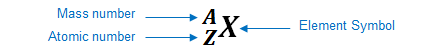# Problem: Part AHow many electrons are in the ion 9Be+?Part BHow many protons are in the ion 9Be+?Part CHow many neutrons are in the ion 9Be+?

###### FREE Expert Solution

We’re being asked to determine the number of electrons, protons, and neutrons in the ion 9Be+

An element can be represented as:You can determine the identity of the element using its atomic number:

Atomic number (Z):

represents the identity of the element
reveals the number of protons
in a neutral element:

# of protons = # of electrons

Mass number (A)

reveals the number of protons and neutrons

mass number = # of protons + # of neutrons

Given:           9Be+

88% (181 ratings)###### Problem Details

Part A

How many electrons are in the ion 9Be+?

Part B

How many protons are in the ion 9Be+?

Part C

How many neutrons are in the ion 9Be+?

Frequently Asked Questions

What scientific concept do you need to know in order to solve this problem?

Our tutors have indicated that to solve this problem you will need to apply the Ions concept. You can view video lessons to learn Ions. Or if you need more Ions practice, you can also practice Ions practice problems.